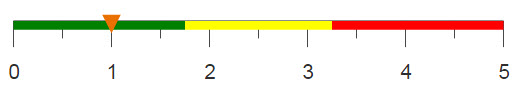# Counting occurrences

Based on specified groups in your data, you can determine how many times pairs of values occur together.

## Difficulty## Objective

You want to know how many times two different products were purchased in the same transaction. You know how to do this in SQL by using SELECT and JOIN, and you would like to produce the same results with 1010data.

## SQL solution

```SELECT COUNT(*)
FROM
(SELECT DISTINCT sku,transid FROM sales_item_detail WHERE sku=406444) AS t1
JOIN
(SELECT DISTINCT sku,transid FROM sales_item_detail WHERE sku=407358) AS t2
ON t1.transid=t2.transid;```

To find the number of transactions that contain both items, you need to perform two different selection statements to get the transaction ID's where each SKU is bought, and join them together on matching ID's. Then, you count the total number of rows to obtain 691 transactions in which both items were bought.

Using 1010data, you can calculate the number of transactions without having to join together multiple results. Additionally, completing this calculation in 1010data is much faster than SQL, especially for larger data sets and more complicated queries.

## 1010data Macro Language solution

```<base table="pub.doc.retail.altseg.sales_detail_transid"/>
<willbe name="first_sku" value="sku=406444"/>
<willbe name="second_sku" value="sku=407358"/>
<sel value="g_or(transid;;first_sku)&g_or(transid;;second_sku)"/>
<tabu label="Tabulation on Sales Detail">
<tcol source="transid" fun="ucnt" name="num_trans"
label="Number of Transactions"/>
</tabu>```

To determine if any one transaction holds either of the desired items, two flag columns are created, called first_sku and second_sku. These columns hold either a 1, if the specified SKU is present, or a 0, if the SKU is not present. The function g_or(G;S;X), uses these columns to determine if each transaction contained at least one of these items. Combining two of these functions in a single select statement allows you determine if both items were bought in a single transaction. G_functions are unique to 1010data, and they allow you to perform calculations much faster because they utilize the unique structure of 1010data which recognizes order. Finally, a tabulation is used to count the number of unique transactions ID's in order to determine how many transactions contained both items.

## Alternate 1010data Macro Language solution

```<base table="pub.doc.retail.altseg.sales_detail_transid"/>
<sel value="g_first1(transid sku;;)"/>
<willbe name="sku_present" value="sku=406444 407358"/>
<sel value="sku_present=1"/>
<tabu label="Tabulation on Sales Detail" breaks="transid">
<tcol source="sku_present" fun="cnt" name="sum_skus"
label="Sum of`SKU's Present"/>
</tabu>
<sel value="sum_skus>1"/>
<tabu label="Number of Transactions with Both Sku's">
<tcol source="transid" fun="cnt" name="num_trans"
label="Number of Transactions"/>
</tabu>```

There is also a way to produce the same results in 1010data without using g_functions. Again, a flag column is created, called sku_present, which holds a 1 if the SKU is present and a 0 otherwise. By performing a tabulation that sums the flag column for each transaction, you can determine if both items were bought together if the sum is 2 or greater. Selecting only these rows and completing a count tabulation results in the number of transactions that contain both specified items.

## Further reading

If you would like to learn more about the functions and operations discussed in this recipe, click on the links below:

g_or(G;S;X)

<tabu>